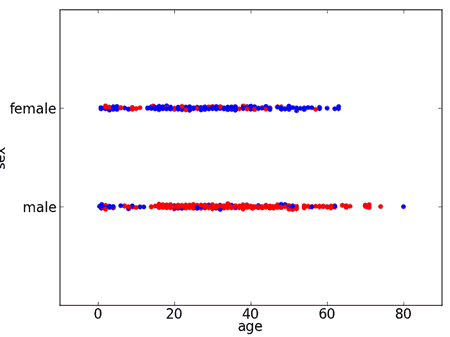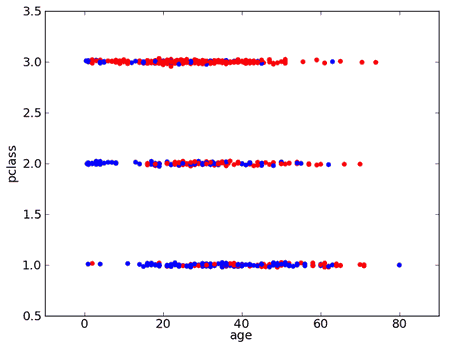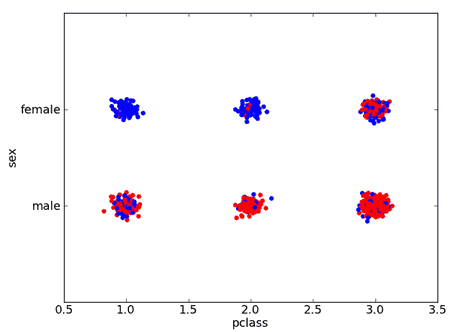# ML Wiki

## Rule-Based Classifier

Rule-based classifiers

• use a set of IF-THEN rules for classification
• if {condition} then {conclusion}
• if part - condition stated over the data
• then part - a class label, consequent

## 1-Rule

### Example

Suppose we have the following dataset

Titanic dataset 
pclass sex age sibsp parch fare embarked survived
3 male 22 1 0 7.25 S 0
1 female 38 1 0 71.28 C 1
3 female 26 0 0 7.93 S 1
1 female 35 1 0 53.10 S 1
3 male 35 0 0 8.05 S 0
3 male 0 0 8.46 Q 0
1 male 54 0 0 51.86 S 0
3 male 2 3 1 21.08 S 0
3 female 27 0 2 11.13 S 1
2 female 14 1 0 30.07 C 1
3 female 4 1 1 16.70 S 1
1 female 58 0 0 26.55 S 1

If we visualize the data by sex

• color-coding survived with blue and not survived with red
•• so we see that most women survived $\to$ can assume that all survived
• e.g. have a rule $x.\text{women} \Rightarrow \text{survived}$

Rules

• there could be many rules like this
• if most children under 4 survived, assume all children under 4 survived
• if most people with 1 sibling survived, assume all people with 1 sibling survided
• calculate misclassification error (error rate) and take the best!

Some variables have more than 2 values

•• if pclass = 1 then survived=yes
• if pclass = 2 then survived=yes
• if pclass = 3 then survived=no

### One Rule Algorithm

• for each attribute $A$
• for each value $V$ of $\text{dom}(A)$
• create a rule
• find the most frequent class $c$
• create a rule "if $A = V$ then $c$
• calculate the error rate of this rule
• select attribute with best error rate for its rules

Can see 1-Rule as a one-level Decision Tree (Data Mining)

• one branch for each value
• each branch assigns most frequent class

## Several Conditions

We also can consider several attributes at the same time

•• IF pclass='1' AND sex='female' THEN survive=yes
• IF pclass='2' AND sex='female' THEN survive=yes
• IF pclass='2' AND sex='male' THEN survive=no
• IF pclass='3' AND sex='male' THEN survive=no
• ...

Can go further

• IF pclass='3' AND sex='female' AND age < 4 THEN survive=yes
• IF pclass='3' AND sex='female' AND age >= 4 THEN survive=no
• IF pclass='1' AND sex='male' AND age < 5 THEN survive=yes

### Getting Rules

Where to extract these rules from?

• from Decision Trees
• each path from top to the bottom is a rule, and the leaf is a class
• sequential covering - for learning rules directly (PRISM algorithm )
• repeatedly removes a portion of the dataset
• the portion - instances covered by the most promising rule at each stage

### Sequential Covering Algorithm

PRISM(dataset $D$):

• $R$ - resulting rule dataset, $R \leftarrow \varnothing$
• for each class $C$
• while $D \not \equiv \varnothing$
• $r \leftarrow$ FindNextRule($C, D$)
• $R \leftarrow R \cup \{ r \}$
• remove from $D$ all instances correctly classified by $r$

Finding the next rule 

• FindNextRule($C, D$) subroutine
• uses Depth-First Search to construct the next rule for class $C$
• we know the consequent for this rule: it's $C$
• so we need to construct only antecedent (предыдущий член отношения)
• iteratively add most promising "attribute=value" constraints
• continue DFS until the rule is specific enough to make no classification errors in the given dataset

FindNextRule(class $C$, dataset $D$):

• let $A$ be all attributes in $D$
• let $r$ be the initial rule $r: \varnothing \to C$
• not examining anything, just always returning $C$
• while $r$ incorrectly classifies some non-$C$ instances in $D$
• let $\text{ant}(r) \to C$ be the rule computed at the previous iteration
• $\text{ant}(r)$ is the antecedent of $r$;
• it means take the rule from the previous iteration of the rule creation loop
• (or an empty rule if this is the first iteration)
• for each pair $(a, v)$ s.t. $a \in A$ and $v \in \text{dom}(a)$
• consider rule $\text{ant}(r) \land (a = v) \to C$
• calculate the accuracy of this rule
• let $(a^*, v^*)$ be the pair with the best accuracy
• so update $r$ by adding this condition:
• let $r: \text{ant}(r) \land (a^* = v^*) \to C$
• remove attribute $a^*$ from $A$:
• $A \leftarrow A - \{ a^* \}$
• return $r$

### Example

A good example of PRISM can be found at 

### Strategies for Learning Rules

General-to-Specific

• add constraints to eliminate negative examples
• stop when only positive examples are covered

Specific-to-General

• remove constraints to cover more positive examples
• stop when further generalization starts covering negatives

## Evaluation

Each rule can be evaluated using these measures

• coverage: # of data points that satisfy conditions
• accuracy = # of correct predictions / coverage

Other measures of "promisingness"